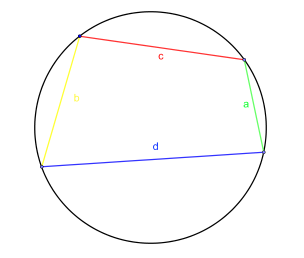#### You may also like### Doodles

Draw a 'doodle' - a closed intersecting curve drawn without taking pencil from paper. What can you prove about the intersections?### Russian Cubes

I want some cubes painted with three blue faces and three red faces. How many different cubes can be painted like that?### Picture Story

Can you see how this picture illustrates the formula for the sum of the first six cube numbers?

# Long Short

##### Age 14 to 16Challenge LevelDraw a circle with radius $1$ unit. Choose any four points on the circumference and join them together to form a quadrilateral.

Label the sides: $a$ is the shortest, $b$ is the next shortest, then $c$, and finally $d$ is the longest side (it is possible to have two sides of equal length).

What is the maximum length that the shortest side $a$ could be?

Side $b$ must be less than a certain value - what value?

What is the maximum length that the longest side $d$ could be? Is it possible for $c$ and $d$ both to be this maximum length?